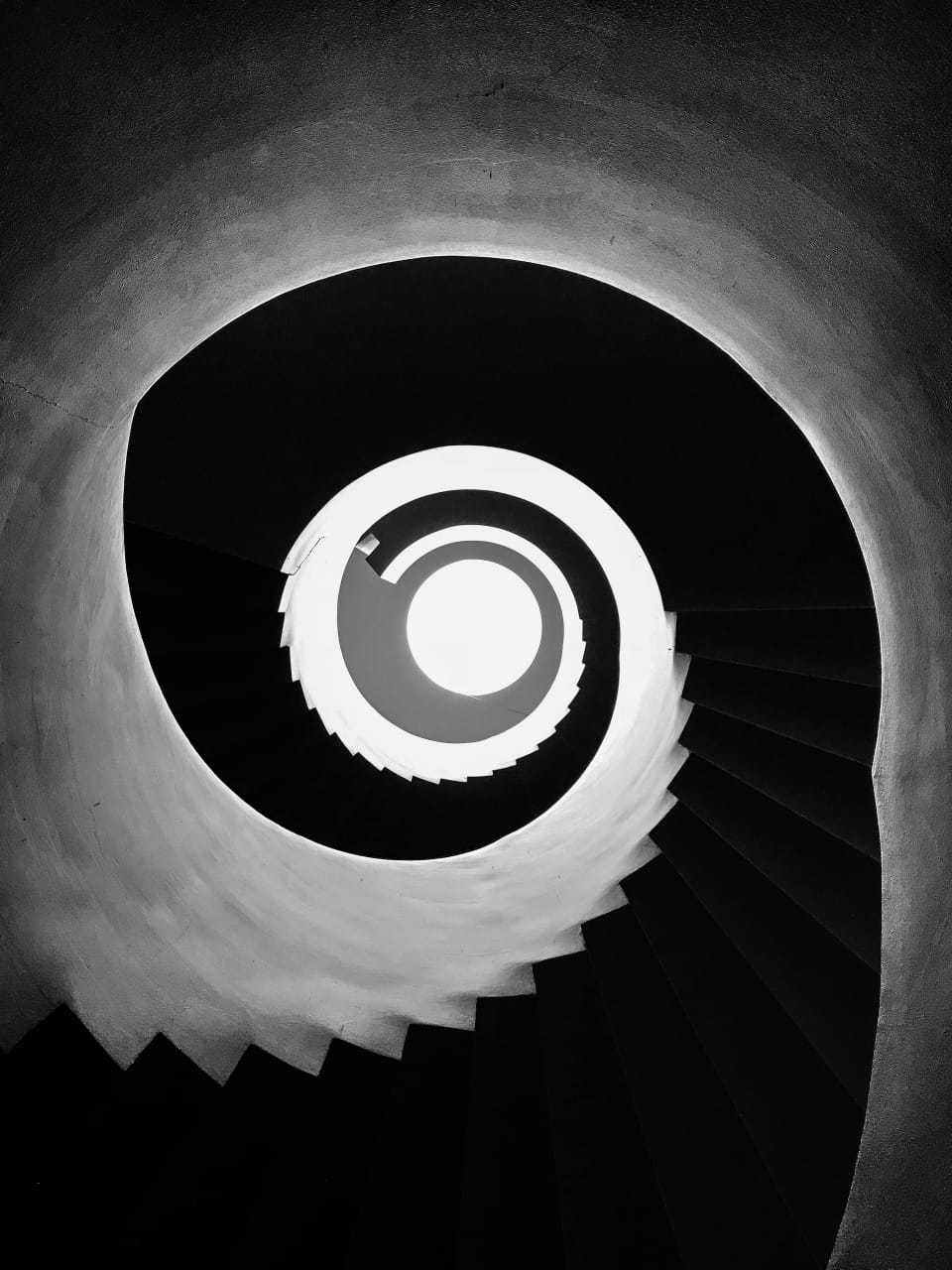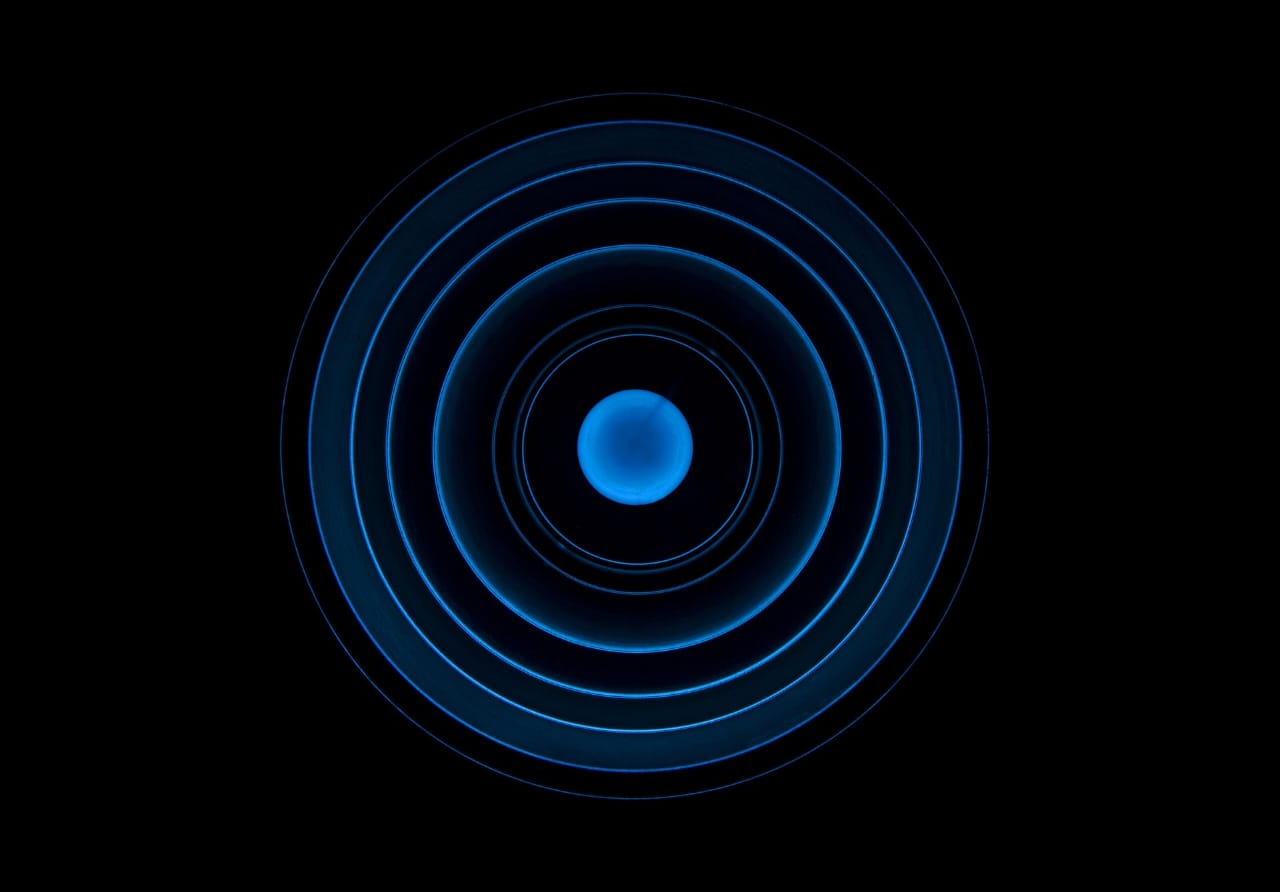What is a circle? What is the diameter of a circle? Also, what is the value of the pie? Here, you will learn about these answers and fun facts about circles.

The circle is the one shape that appears in the art and architecture of every ancient civilization. It’s not hard to find a tale that involves a circle. Latin “Circulus,” meaning “little ring,” is where we get our term “circle.”

Since it only exists in two dimensions, a circle has no corners or edges. We see a circle forming mathematically when a point moves in a plane at a constant distance.

It may make you think of Geometry class and even make you feel a little scared, but I promise not to bore you with the details. Here are some facts about circles you may not know about. So, let’s find out:

1. A circle is a flat, two-dimensional shape made up of a collection of points all the same distance from a central origin.

2. No other shape with only one side can contain an area like a circle. A circle with an infinite diameter is a straight line.

3. Mathematically speaking, a circle only refers to the outermost part of a shape, while the term “disk” encompasses the entire thing.

4. For as long as we’ve been around, we’ve been able to recognize circles. The Sun and Moon, the human eye, and shell shapes all form a circle because they are naturally occurring.

5. The wheel (a circular object) was one of the most significant innovations in human history.

6. The diameter, conceptualized as a line segment that traverses a circle’s center, represents the maximum allowable straight-line distance between the two points.

7. Archimedes first demonstrated that the area contained by a circle is equivalent to a triangle’s area whose base is similar to the circle’s circumference and height of the circle’s radius.

8. Due to the inherent reflection symmetry of its many lines of symmetry, the circle is the most symmetrical shape. Every angle around its center is identical when rotated.

9. The ratio of a circle’s circumference to its diameter is Pi, an irrational number. Roughly speaking, it equates to 3.14.

10. The Rhind papyrus, dated to around 1700 BCE, provides instructions for computing the surface area of a circle. A rough estimate of the answer is 3.16, which we derive by dividing 256 by 81. (close to pi).11. The incircle is a specific kind of circle you can draw within any triangle if all three sides are perpendicular to the circle.

12. The area of a circle is infinite. It also has a straight line. You can make out some additional symmetry lines.

13. We call the angle formed when a line touches a circle at any one of its points a tangent angle (point of tangency). Its angle with the circle’s radius is always 90 degrees.

14. When the secant and its exterior part overlap an endpoint beyond the circle, the product of their lengths is equal to the square of the length of the tangent segment.

15. When ants lose their pheromone trail, they herd together and go in circles until they become exhausted and die.

16. When the two foci of an ellipse are at the same place, and the eccentricity is zero, we have a circle.

17. The arc of a semicircle, which is half of a circle, is 180 degrees.

18. It would appear that circles are a symbol of the divine in many cultures. Circular or almost circular shapes occur in nature in other locations as well.

19. It is mathematically proven that a circle will have no angles. You can find real-circle examples of circles in the form of flat plates, coins, and tires.

20. Only when the two sets of angles are complementary can a quadrilateral be inscribed inside a circle.21. It is well-known that the Greeks held the Egyptians to be the original developers of geometry.

22. The diameter (d) is the distance between the centers of two points on a circle.

23. Animal behavior is likewise classified according to the revolving circle. An army of ants is visible here. When they become separated in pheromone tracks, they will eventually die of weariness.

24. People don’t have a built-in ability to find their way around the world. Due to the absence of directional cues, they end up walking in a never-ending circle.

## Conclusion

I’m sure the facts mentioned in this article are new to you. We don’t get to learn many things in our schools and colleges. These facts will help you broaden your knowledge of the various circles.